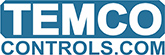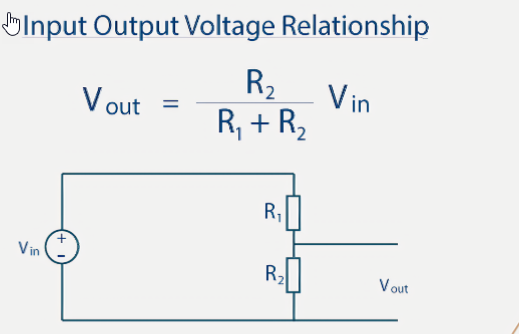# Fluid Level Sensor

Hi Maurice,

reviving this post, I received a 10k floater and a liquid level sensor and I tried to hook them.to a T3.
The liquid level sensor is a 0.10V unit and the result is enough sensitive: I obtained nice curves of the voltage variations.

However, despite the fact that the floater is a 10k resistive unit (I tested with an ohmmeter and the results is accurate: from 0 to 10k ohm by 1k steps), I tested a T3 without positive results: selected as a 10K temp sensor (…) or created a custom table with no real results. I can’t retrieve the resistance values.
I don’t need to convert values, I’ll be glad if I can obtain values (ohms), my plc will do the maths.
Thank you

Here’s the chart of resistance versus liquid level for the 10k float sensor we have in our web store.

The voltage at the input terminal of the controller can be calculated using the voltage divider formula. When the input is configured for dry contact/thermistors there is a weak excitation voltage provided through a 10k resistor tied to 3V. So the resistance can be calculated by the formula:
V out = R2 / ( R2 + 10,000 ) * 3
Where R2 is the value of your resistive sensor or in this case the float level sensor.And finally, build the custom table in the T3000 range settings at Tab2. The line is a straight (albeit stepped) line from the minimum of 0V to the maximum of 1.5V so we only need to enter in the first and last data points at Tab3. We can put the units in at Tab4. Finally, notice at Tab5 that the signal type is set to ‘Thermistor/Dry Contact’

1 Like